# Calculate Decimal Part of Number – Excel & Google Sheets

This tutorial will demonstrate how to calculate the decimal part of a number in Excel and Google Sheets.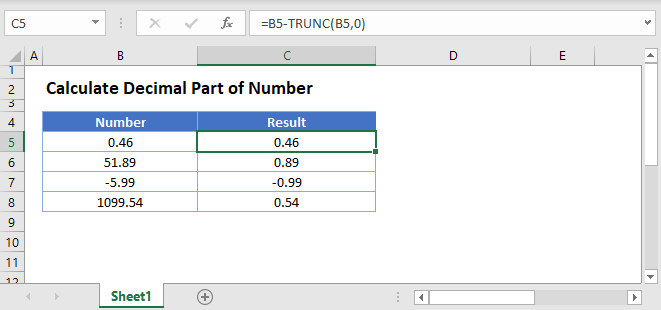## Calculate Decimal Part of Number

### Calculate Integer

To calculate the decimal part of the a number, first we must calculate only the integer part. We can calculate the integer portion of a number using the TRUNC Function.

``=TRUNC(B3,0)``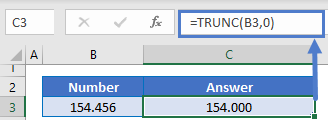You might also be familiar with the INT Function. The INT Function returns the integer portion of the number, which for positive numbers it the same as the TRUNC Function. However, for negative numbers, the INT Function rounds negative numbers down away from zero.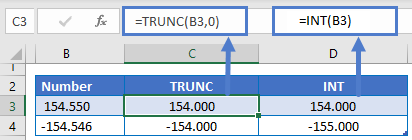For this purpose, you probably want to use the TRUNC Function.

### Calculate Decimal

Next, simply subtract the integer value found above, from the number to calculate only the decimal value.

``=B3-TRUNC(B3,0)``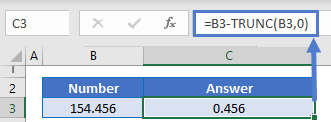## Calculate Decimal Part of Number in Google Sheets

All of the above examples work exactly the same in Google Sheets as in Excel.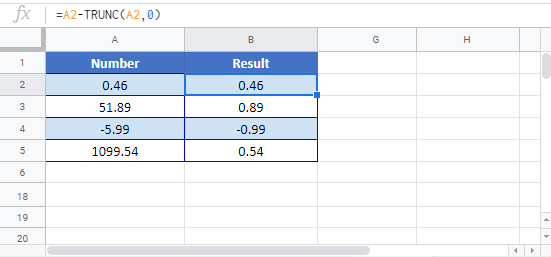### Excel Practice Worksheet

Practice Excel functions and formulas with our 100% free practice worksheets!